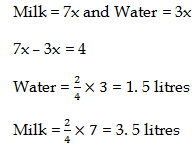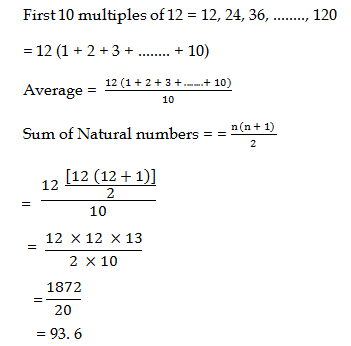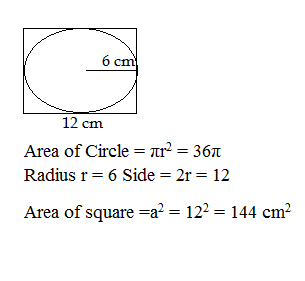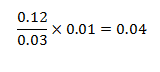# SSC CGL 2018 Practice Test Papers | Quantitative Aptitude (Day-60)

Dear Aspirants, Here we have given the Important SSC CGL Exam 2018 Practice Test Papers. Candidates those who are preparing for SSC CGL 2018 can practice these questions to get more confidence to Crack SSC CGL 2018 Examination.

[WpProQuiz 3751]

Click “Start Quiz” to attend these Questions and view Explanation

1) 411 + 412+ 413 + 414 is divisible by

a) 13

b) 17

c) 19

d) 11

2) For what value of K, 313K will be a perfect square, where 313K is a 4 digit number?

a) 2

b) 4

c) 6

d) 1

3) Fahim is 10% more efficient than Faruk. If Fahim can complete a piece of work in 33 days, then Faruk can complete the same work in how many days?

a) 35 days

b) 44 days

c) 45 days

d) 30 days

4) After giving 10% on an article there is a profit of 10%. What will be the profit percentage when no discount is given?

a) 11%

b) 12%

c) 21%

d) 10%

5)  What is the value of

a) 54/5

b) 94/12

c) 90/7

d) 84 / 5

6) If 50% of a number is added to 100, then the result is the number itself, then what is the number?

a) 100

b) 200

c) 400

d) 800

7) A mixture contains Milk and water in the ratio of 7 : 3. If it contains 2 litres more water than milk, then what will be the quantity (in litres) of water in the mixture?

a) 1.5

b) 3.5

c) 2.5

d) 4.5

8) What is the average of first 10 multiples of 12?

a) 86. 2

b) 93.6

c) 74.2

d) 69. 8

9) An Area of circle which is inscribed in a square is 36π cm2. What is the area of Square (in cm2)?

a) 64

b) 81

c) 144

d) 49

10) What is the value of 0.12 ÷ 0.03 × 0.01?

a) 0.4

b) 0.04

c) 4

d) 0.0004

411 + 412+ 413 + 414

= 410 (41 +42 + 43 + 44)

= 410 (4 + 16 + 64 + 256) = 410 × 340

∴ 340 is divisible by 17

3136 = 562

Fahim = 1/33

Faruk = 110x/100

= 33 × 100 / 110 = 30 days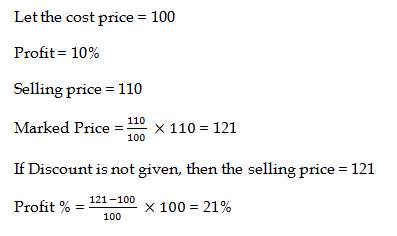50% of x + 100 = x

50x/100 + 100 = x

x+ 200 /2 = x

x + 200 = 2x

x = 200